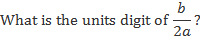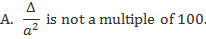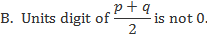Alternatively, you can register/login faster using

Register Free!
 QUESTION OF THE DAY
Question of the day
HOME > MBA PREP CENTRE > DAILY TEST PREP > QUESTION OF THE DAYSee More Questions
Question of the Day (30-Oct-19)

 Views : 2793 Rated 4.8 by 20 Users
A question is followed by two statements, A and B. Answer the question using the following instructions:

Mark (1) if the question can be answered by using the statement A alone but not by using the statement B alone.

Mark (2) if the question can be answered by using the statement B alone but not by using the statement A alone.

Mark (3) if the question can be answered by using either of the statements alone.

Mark (4) if the question can be answered by using both the statements together but not by either of the statements alone.

Mark (5) if the question cannot be answered on the basis of the two statements.

Let p and q be the two distinct roots of the quadratic equation ax2 + bx + c = 0. Both p and q are two digit integers with same units digit. Let ∆ be the determinant of this equation.OPTIONS 1) 1 2) 2 3) 3 4) 4 5) 5